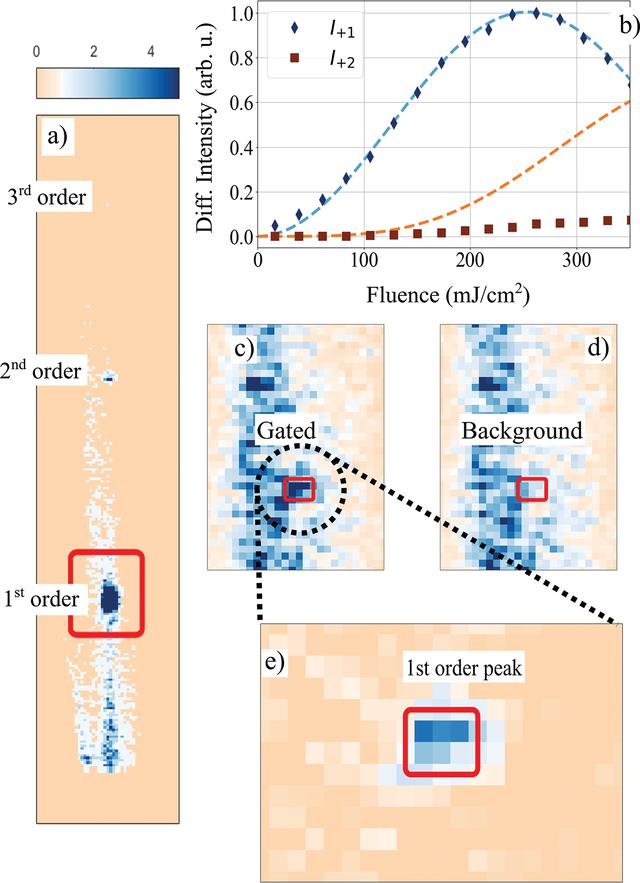disable zoom     view article Figure 4 Synchrotron pulse gating. (a) Detector image depicting diffraction from a single synchrotron pulse in the first, second and third diffraction order. The pixel size is 55 µm × 55 µm, the red square marks the area (25 × 35 pixels) which is shown in (c) and (d). (b) Fluence dependence of the maximum diffracted intensity in the first (black diamonds) and second (brown squares) diffraction order. The dashed lines show calculated intensity using equation (1). The intensity of the first and the second order do not have the predicted ratio. (c, d) Diffraction in the first order with an external detector gate width of 50 µs, i.e. averaging 255 X-ray pulses from the synchrotron. Both images show the region marked with a red square in (a) and use the same colorscale. The long streak stems from diffuse surface scattering. The first diffraction order in the gated [(c)] and background [(d)] image is marked by the red rectangle. (e) Background-corrected image (20 × 12 pixels) of the first-order diffraction peak. The image was produced by subtracting the images (c) and (d).JOURNAL OFSYNCHROTRONRADIATION
ISSN: 1600-5775
Volume 28| Part 2| March 2021| Pages 375-382